# Precalculus : Find the Area Using Limits

## Example Questions

### Example Question #1 : Find The Area Using Limits

What is the area under the curve of the function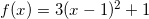fromto.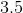Explanation:

The area under the curve of the functionis the definite integral fromto.

Remember when integrating, we will increase the exponent by one and then divide the whole term by the value of the new exponent.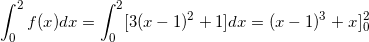From here, we find the difference between the function values of the boundaries.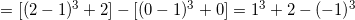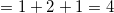### All Precalculus Resources